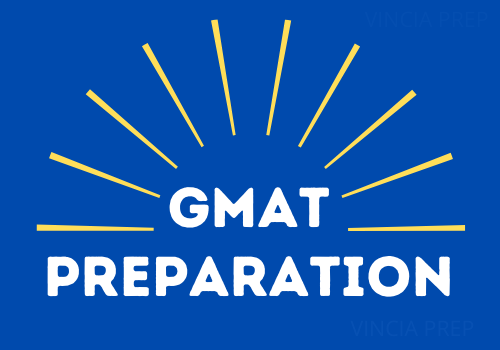Introduction into Data Sufficiency for the GMAT

## TestimonialI was recently admitted to Boston College with a \$41,000 scholarship! I am sure that without your support it would not have been possible!

# Introduction into Data Sufficiency for the GMATWhen you first start studying the math section on the GMAT you'll run up against these weird data sufficiency (DS) questions. Before you start doing these strange problems you need to know their different parts and what they are asking you to do!

A data sufficiency question looks like this:

If x is less than 3 then what is the value of x ?  <---------- Question stem.  Here they often give you not only the question, but also some information that can help you solve the problem.

1. x  is a prime number. <------------------------------------------Statement 1.
2. x + 3 equals the largest prime factor of 30<--------------- Statement 2.

What data sufficiency questions are asking for is whether the information in the statements are enough to answer the initial question.  This means, if you can give an exact number, say yes, or say no the answer sufficient.

So here, we first look at statement one.  If x is a prime number do you know the value of x ?  As it turns out, you can say what value x is because in the question stem we are told that x < 3.  Since the first prime number is the number "2", and the next prime number is "3" and since x < 3 we can say that x must equal 2.  Since we can give an exact answer for x, statement 1 is sufficient.    If we had found that x could equal 2 and some other number the statement would not be sufficient.

Next we look at statement two.  To start we need to know what prime factors are.  If you don't know, don't worry.  When you go through a strategy book or a gmat class it'll be explained.

For now, just know that the prime factors of 30 are 2, 3, and 5.  So the largest prime factor of 30 is 5.

Statement 2 says  x + 3 equals the largest prime factor of 30 so we can put in our new information and say

x + 3 = 5.  Therefore, x must equal 2.  Thus, this statement is also sufficient.

Next we need to look at the answer choices.  The answers are:

A) Statement 1 alone is sufficient but statement 2 alone is not sufficient to answer the question asked.

B) Statement 2 alone is sufficient but statement 1 alone is not sufficient to answer the question asked.

C) Both statements 1 and 2 together are sufficient to answer the question but neither statement is sufficient alone.

D) Each statement alone is sufficient to answer the question.

In our question we found that each statement was sufficient so the answer is D.

If statement 1 had been sufficient and statement 2 was not sufficient then answer would have been A.

If statement 2 had been sufficient and statement 1 was not sufficent then the answer would have B.

Sometimes we need the information in Statement 1 and Statement 2 to answer the question.  For example if we had the question

X = ?

1) x is even

2) x is prime

For statement 1 the response could be 2, 4, 6, etc... and for statement 2 the response could be 2, 3, 5, 7, 11 etc... Therefore, individually, each statement is not sufficent since they both have more than one possible value for x.  However, if we take the two statements together, X = 2.  Since we can find the answer if we use both statements, the correct answer choice is C.

Lastly, there are times when with all of the information in the question stem, and both statements we still can't find one exact answer.  In these cases, the answer is E.For more information about how to start working on Data sufficiency questions you can check out our video:If you need help studying for the GMAT, contact Dan now.  In order to provide the maximum amount of attention to everyone, only 10 students a month can be accepted.
 Emaildan@vinciaprep.comPortable+33 (0)6 41 75 45 71Main Office Address5 rue Legraverend 75012Secondary offices